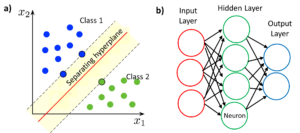# NIR spectroscopy and Machine Learning

Near infrared spectroscopy (NIR), using commonly halogen white light sources, is employed in the agrifood industry to recover information about the chemical composition and quality parameters of different products in a non-invasive, fast and accurate way, providing an advantage over conventional measuring methods, as in the case of fruit and  vegetables, wine or meat.

However, the relationships between the spectral measurements and the quality parameters are not trivial, and different machine learning methods are employed for modeling them. In this post, an overview of the commonly employed calibration methods based on machine learning will be provided.

There are two main types of machine learning algorithms: supervised learning algorithms and unsupervised learning algorithms. There are also semi-supervised learning algorithms, which are a mixture of both. In the case of supervised learning algorithms, the algorithms are “taught” by a human the outputs that correspond to certain inputs (these pairs are called labeled data) so they can predict the outputs that correspond to new inputs. These algorithms can have two purposes: classification, that is, organizing products or objects into different classes depending on their characteristics; or regression, that is, recovering the value of a certain property such as the concentration of a biochemical parameter (proteins, lipids, sugars).

In the case of unsupervised learning algorithms, there is no human intervention. The algorithms try to detect patterns based on a set of unlabeled data. These algorithms tend to be useful when the human does not know what to look for in the data. There are two main types of algorithms: clustering algorithms, whose purpose is classifying the data into groups according to its similarity; and association algorithms, whose objective is finding rules within the data set.

In the following paragraphs, the basics of four machine learning algorithms are described: Principal Component Analysis (PCA), Support Vector Machine (SVM), Partial Least Squares (PLS)  and Artificial Neural Networks (ANNs).

Principal Component Analysis (PCA) is an unsupervised method that reduces the dimensionality of the initial data but preserves the variance. The initial data are transformed into independent variables named principal components (PCs) where each component is a linear combination of the original data. The number of PCs is equal to the number of original variables, but early PCs explain most of the variance, which allows the dimensionality reduction. A PCA enables to show the inherent structure of the data.

Support Vector Machine (SVM) is a widely employed supervised algorithm, used for classification of the samples. The purpose of this algorithm is to find a hyperplane in an N-dimensional space (being N the number of variables) that separately classifies two sets of data points. If the number of variables is 2, the hyperplane is a line; and if the number of variables is 3, the hyperplane is a two-dimensional plane.  The best hyperplane for a given case is the one that obtains the maximum margin between the two classes. SVM algorithms use kernel functions to transform the original space into a higher dimensional space where the class separation is easier. SVM can also be adapted for multiclass (more than 2 classes) classification.Figure 1. a) Data classification by Support Vector Machine algorithm. b) Basic schema of a neural network.

Partial Least Squares (PLS) is a method for developing predictive models when there is a huge number of variables (factors) and these are highly collinear. PLS employs information from all wavelengths in the entire NIR spectrum to predict sample composition, instead of utilizing a few selected wavelengths. The objective of PLS is to use the factors to predict the responses. This is achieved indirectly by extracting latent variables T and U from sampled factors and responses respectively. The extracted variables T (also named X-scores) are employed to predict the Y-scores (U) and these Y scores are used to predict the responses.

An Artificial Neural Network (ANN) is a machine learning model that mimics the operation of neurons in a biological brain. The neural network contains nodes (neurons) organized in several layers. There is an input layer and an output layer that can be directly connected through one or more hidden layers. The output of each neuron is calculated as a non-linear function of the sum of its inputs, and it is sent to all nodes in the next layer after applying a coefficient or weight. These weights are adjusted through the training of the neural network. The main advantages of neural networks are that they do not require the development of an explicit model and that the failure of one of the neurons does not affect the operation of the system.

In conclusion, the combination of NIR spectroscopy with machine learning algorithms plays a key role in the consolidation of NIR spectroscopy as a non-invasive, non-destructive and fast tool for food quality analysis. Some of the most widely known algorithms have been described in this post although many more exist.

Written by J.J. Imas

Bibliography

 M. Zareef, Q. Chen, M. M. Hassan, M. Arslan, M. M. Hashim, W. Ahmad, F. Y. H. Kutsanedzie, and A. A. Agyekum, “An Overview on the Applications of Typical Non-linear Algorithms Coupled With NIR Spectroscopy in Food Analysis,” Food Eng. Rev. 12(2), 173–190 (2020).

 “All About ANN – Artificial Neural Networks and Chemometric Modeling for NIR Spectroscopy | Tools for Applied Food Science” https://felixinstruments.com/blog/all-about-ann-artificial-neural-networks-and-chemometric-modeling-for-nir-spectroscopy/.

 P. S. Sampaio and C. M. Brites, “Near-Infrared Spectroscopy and Machine Learning: Analysis and Classification Methods of Rice,” Integr. Adv. Rice Res. (2021).

 “Types of Machine Learning Algorithms You Should Know | by David Fumo | Towards Data Science,” https://towardsdatascience.com/types-of-machine-learning-algorithms-you-should-know-953a08248861

 “Support-vector machine – Wikipedia,” https://en.wikipedia.org/wiki/Support-vector_machine

 “An Introduction to Partial Least Squares Regression,” R. D. Tobias, Proceedings of the twentieth annual SAS users group (1995)

 “Artificial neural network – Wikipedia,” https://en.wikipedia.org/wiki/Artificial_neural_network